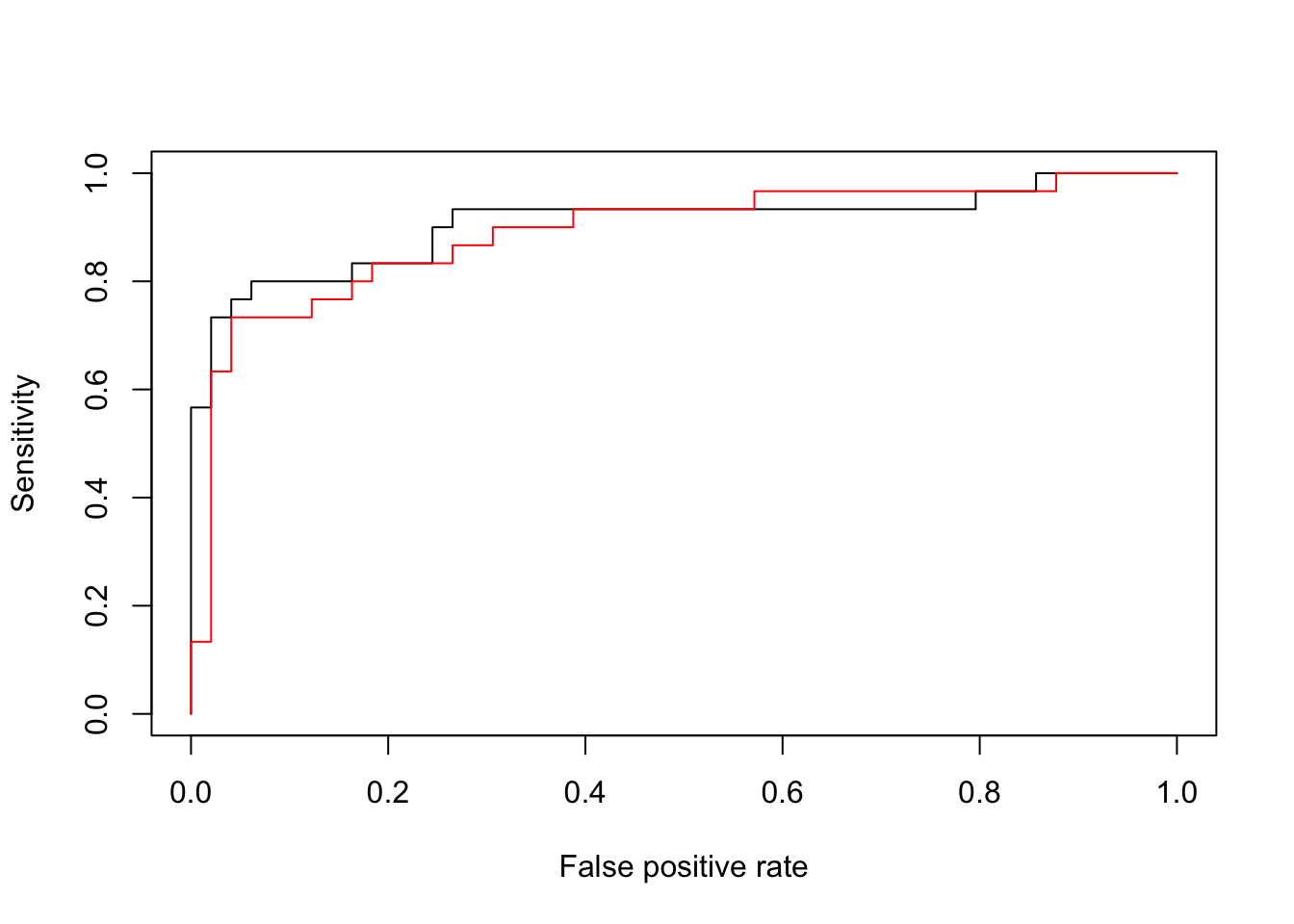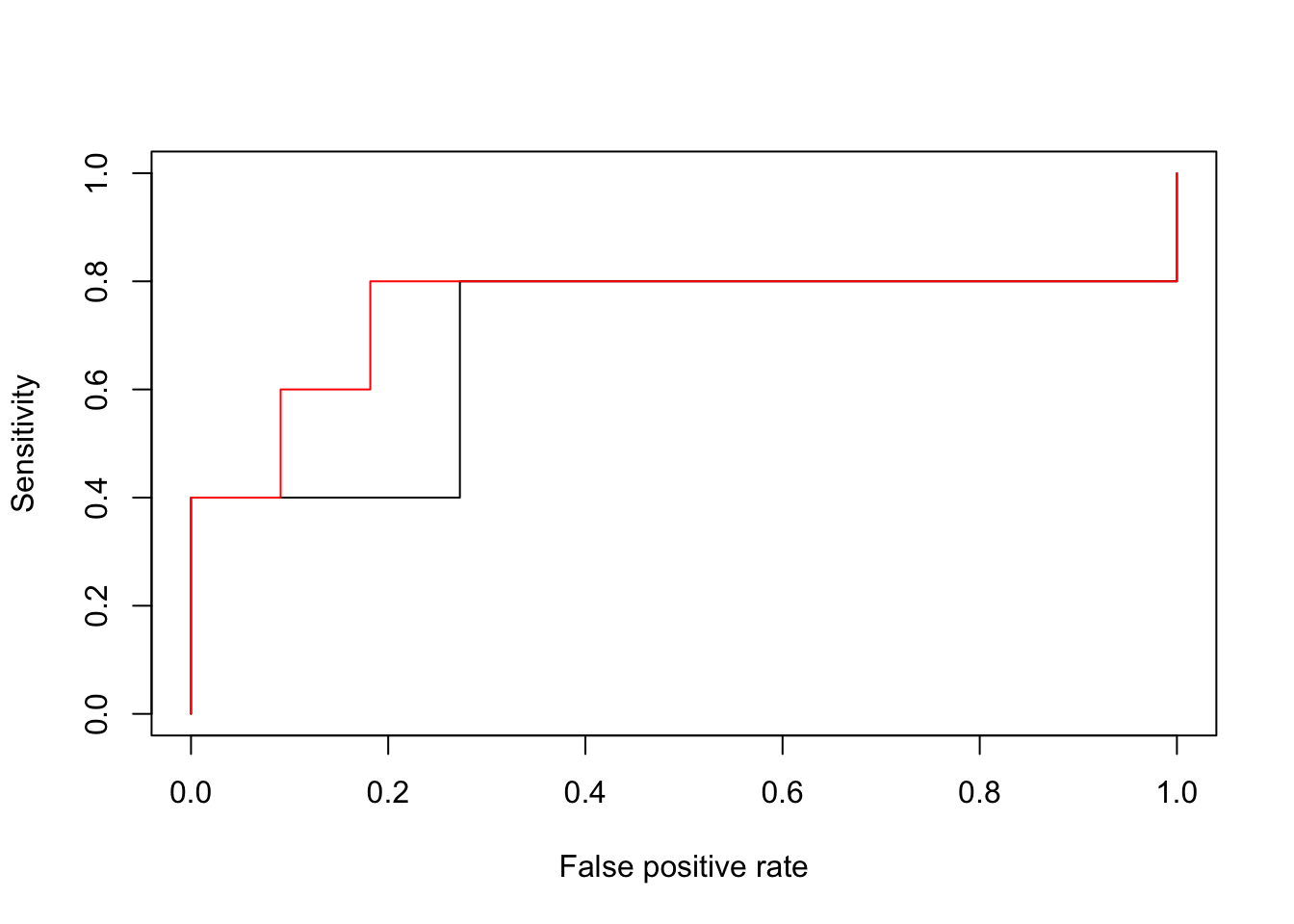# Example: Classification for endometrial cancer

Endometrial <- read.table("Endometrial.dat", header = T)
Endometrial
• HG: histology grade (0: low / 1: high)
• NV: neovasculation
• PI: pulsatility index
• EH: endometrium height

## 1.1 Quasi-complete seperation

table(Endometrial$NV, Endometrial$HG)
##
##      0  1
##   0 49 17
##   1  0 13

If $$NV_i > 0$$, then $$y_i = 1$$. If $$NV_i = 0$$, then $$y_i$$ can be either $$0$$ or $$1$$.

We can see that R returns very large parameter estimation

fit <- glm(HG ~ NV + PI + EH, family = binomial, data = Endometrial)
summary(fit)
##
## Call:
## glm(formula = HG ~ NV + PI + EH, family = binomial, data = Endometrial)
##
## Deviance Residuals:
##      Min        1Q    Median        3Q       Max
## -1.50137  -0.64108  -0.29432   0.00016   2.72777
##
## Coefficients:
##               Estimate Std. Error z value Pr(>|z|)
## (Intercept)    4.30452    1.63730   2.629 0.008563 **
## NV            18.18556 1715.75089   0.011 0.991543
## PI            -0.04218    0.04433  -0.952 0.341333
## EH            -2.90261    0.84555  -3.433 0.000597 ***
## ---
## Signif. codes:  0 '***' 0.001 '**' 0.01 '*' 0.05 '.' 0.1 ' ' 1
##
## (Dispersion parameter for binomial family taken to be 1)
##
##     Null deviance: 104.903  on 78  degrees of freedom
## Residual deviance:  55.393  on 75  degrees of freedom
## AIC: 63.393
##
## Number of Fisher Scoring iterations: 17

You can still run a likelihood-ratio statistics with df = 1 for $$H_0$$: $$\beta_1 = 0$$

deviance(glm(HG ~ PI + EH, family = binomial, data = Endometrial)) - deviance(fit)
##  9.357643

Compare with a Chi-square statistics, the p-value is 0.002. We can conclude that beta1 > 0. We will present other inferences in later sections.

We can also run the deviance analysis

fit1 <- glm(HG ~ PI + EH + NV, family = "binomial", data = Endometrial)
anova(fit1, test = "LRT")

## 1.2 ROC curve

Here we used all samples to train the logistic regression, and we now look at the training error with ROC curve.

Endometrial$pred <- predict(fit, Endometrial, type = "response") Endometrial library(ROCR) pred <- prediction(Endometrial$pred, Endometrial$HG) perf <- performance(pred,"sens","fpr") plot(perf) ## Compare with a simplier model fit2 <- glm(HG ~ PI + EH, family = "binomial", data = Endometrial) Endometrial$pred2 <- predict(fit2, Endometrial, type = "response")
pred2 <- prediction(Endometrial$pred2, Endometrial$HG)
perf2 <- performance(pred2,"sens","fpr")
plot(perf2, add = T, col = "red")We see a slighted larger error for the simplier model.

For an unbiased evaluation of the classification performance, we should look at the ROC curve on a test dataset. We can also randomly select $$20\%$$ samples as test datae.

n <- nrow(Endometrial)
set.seed(1)
test.idx <- sample(n, round(0.2*n))
fit.train <- glm(HG ~ PI + EH + NV, family = "binomial", data = Endometrial[-test.idx,])
test <- Endometrial[test.idx, ]
test$pred <- predict(fit.train, test, type = "response") pred <- prediction(test$pred, test$HG) perf <- performance(pred,"sens","fpr") plot(perf) ## Compare with a simplier model fit.train2 <- glm(HG ~ PI + EH, family = "binomial", data = Endometrial[-test.idx,]) test$pred2 <- predict(fit.train2, test, type = "response")
pred2 <- prediction(test$pred2, test$HG)
perf2 <- performance(pred2,"sens","fpr")
plot(perf2, add = T, col = "red")We see that the simpler model is better in terms of classification. Anyway, the sample size in this dataset is small, so the randomness in the test data ROC curve is non-negligiable.